• 离散傅里叶级数，离散傅里叶变换逆傅里叶变换的实现。
• matlab傅里叶变换及逆变换和快速傅里叶变换，通过编程实战掌握具体应用。包括matlab傅里叶变换、matlab傅里叶逆变换、matlab快速傅里叶变换。matlab matlab安装教程 matlab下载 matlab图像处理
• 精通matlab傅里叶变换及逆变换和快速傅里叶变换matlab 编程语言 Matlab
• 傅里叶变换及逆变换，C++实现（改成c也可以，没有用到面向对象）
• OpenCV傅里叶变换及逆变换实现代码，机器视觉使用
• 傅里叶逆变换-&gt;读取图像 int main() { cv::Mat img = cv::imread("lena.jpg"); DFTtransform(img); cv::imshow("DFT img", img); cv::waitKey(); system("pause"); ...
代码步骤：
读入图像->傅里叶变换->傅里叶逆变换->读取图像

int main()
{
DFTtransform(img);
cv::imshow("DFT img", img);
cv::waitKey();
system("pause");
return 0;
}
//DFTtransform实现
void DFTtransform(cv::Mat& image)
{
image.convertTo(image, CV_32F);
std::vector<cv::Mat> channels;
split(image, channels);  //分离RGB通道
cv::Mat image_B = channels;
//expand input image to optimal size
int m1 = cv::getOptimalDFTSize(image_B.rows);  //选取最适合做fft的宽和高
int n1 = cv::getOptimalDFTSize(image_B.cols);
//填充0
cv::copyMakeBorder(image_B, padded, 0, m1 - image_B.rows, 0, n1 - image_B.cols, cv::BORDER_CONSTANT, cv::Scalar::all(0));
cv::Mat complexI;
cv::merge(planes, 2, complexI);  //planes, planes是实部和虚部

cv::dft(complexI, complexI, cv::DFT_SCALE | cv::DFT_COMPLEX_OUTPUT);
cv::split(complexI, planes);

//定义幅度谱和相位谱
cv::Mat ph, mag, idft;
cv::phase(planes, planes, ph);
cv::magnitude(planes, planes, mag);  //由实部planes和虚部planes得到幅度谱mag和相位谱ph

/*
如果需要对实部planes和虚部planes，或者幅度谱mag和相位谱ph进行操作，在这里进行更改
*/

cv::polarToCart(mag, ph, planes, planes);  //由幅度谱mag和相位谱ph恢复实部planes和虚部planes
cv::merge(planes, 2, idft);
cv::dft(idft, idft, cv::DFT_INVERSE | cv::DFT_REAL_OUTPUT);
image_B = idft(cv::Rect(0, 0, image.cols & -2, image.rows & -2));
image_B.copyTo(channels);
merge(channels, image);
image.convertTo(image, CV_8U);
}

展开全文• VS2010下实现的离散傅里叶变换和离散傅里叶逆变化代码。
• 我们已经知道一维噪声可以用一维傅里叶变换到频域滤波，同理二维噪声也可以用二维傅里叶变换到"频率滤波"。 二维傅里叶变换的原理 笔者很讨厌一上来就看到一连串复杂的公式！因此当我看懂一个原理后，我就会用最好...
前言
在野外数据采集中，虽然单个仪器采集的是一维信号，但是当把多台仪器数据汇总并生成做二维剖面的图像时，噪声可不只有一维的，更有x,y两个方差同时存在的"二维噪声"！我们已经知道一维噪声可以用一维傅里叶变换到频域滤波，同理二维噪声也可以用二维傅里叶变换到"频率滤波"。
二维傅里叶正变换的原理
笔者很讨厌一上来就看到一连串复杂的公式！因此当我看懂一个原理后，我就会用最好理解的方式来重述它，毕竟我更偏重于应用。二维傅里叶变化，只用一个公式：
公式1：二维傅里叶变换公式(其实不用看它)
公式中参数说明：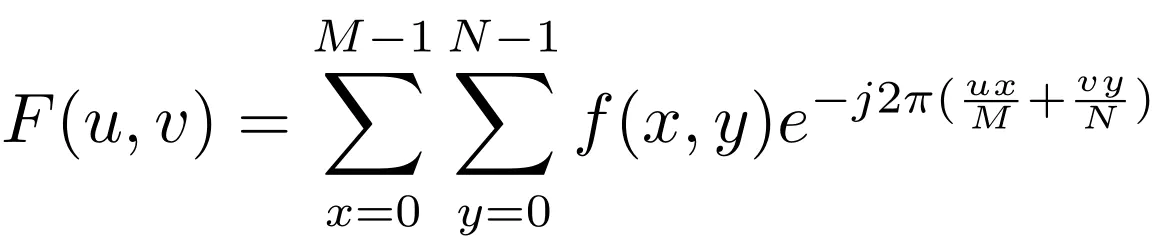公式(1)
公式中参数说明：
M、N分别是图像的长和宽；
u、x范围从1到M-1；v、y范围从1到N-1。
公式看上去不难，但其实还是不太明确到底怎么用啊！它其实可以矩阵相乘的形式表示：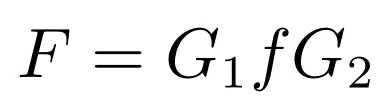公式(2)
公式2中f是原始二维数据矩阵，G_{1}和G_{2}分别是如下：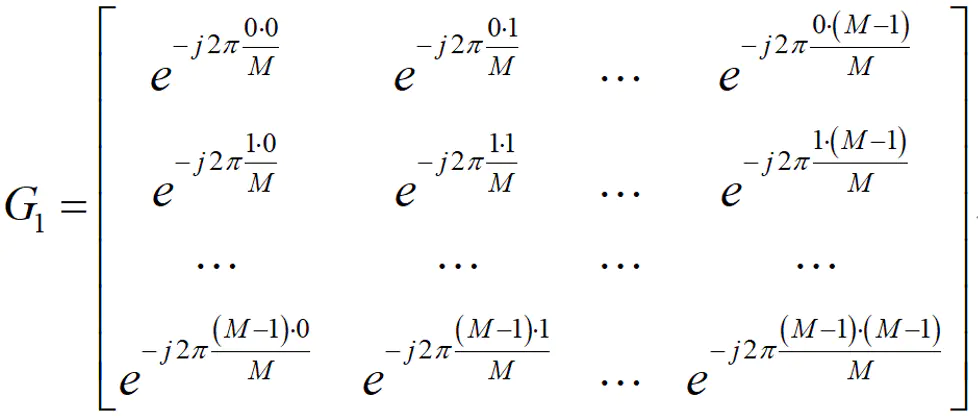公式(3)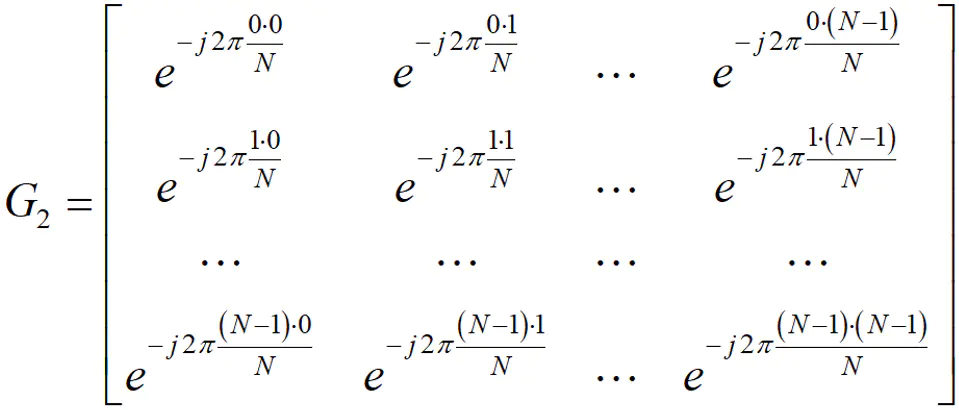公式(4)
这用Matlab太好实现了叭！G_{1}和G_{2}这么有规律，很容易就编程出来了~ 然后3个矩阵做个乘积，就换到频率域了！二维傅里叶变换就结束了！
二维傅里叶反变换
定义的公式为公式5(不用看)，矩阵形式的公式为公式6(看这个即可)：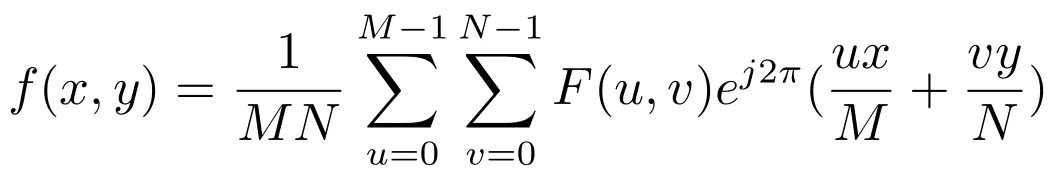公式5：二维傅里叶反变换公式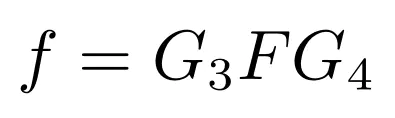公式6：矩阵形式(看这个)
其中G_{3}和G_{4}矩阵为：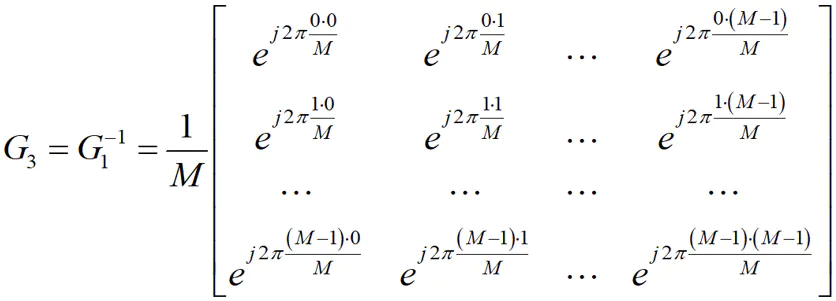公式(7)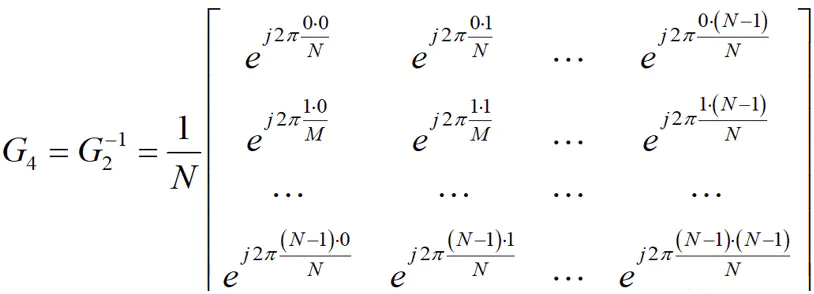公式(8)
到此，二维傅里叶逆变换也结束了！整个二维傅里叶变换就都结束了！真的很简单！下面我们就在Matlab中手写实现正、逆这两个过程。
Matlab程序实现
首先实现正变换程序，对应Matlab自带函数为：fft2
clc; clear;

data = im2double(data);
data = rgb2gray(data);     % rgb转为灰度图像
subplot(1,3,1);
imshow(data);
title('原始图像')

zidai = fft2(data);   % matlab自带函数，来用对比
subplot(1,3,2);
imshow(real(zidai));  % 一般只要实部
title('自带的fft2生成的"频域"图像');

size_data = size(data);
M = size_data(1);  % 图(原始数据矩阵)的长
N = size_data(2);  % 图(原始数据矩阵)的宽

% 下面是傅里叶正变换必备的一些矩阵:
Wm = exp(-j*2*pi/M);
Wn = exp(-j*2*pi/N); % 不同G中用不同的W
Em = zeros(M);
En = zeros(N);     % E是辅助计算矩阵
Gm = zeros(M)+Wm;
Gn = zeros(N)+Wn;  % G是计算时要用的矩阵
F = zeros(M,N);    % F是转换到频域的结果

% 对Gm的计算: 循环长度为M
fprintf('二维离散傅里叶变换开始:\n');
for row = 0:M-1
for col = 0:M-1
Em(row+1,col+1) = row * col;
Gm(row+1,col+1) = Gm(row+1,col+1)^Em(row+1,col+1);
end
end
% 对Gn的计算: 循环长度为N
for row = 0:N-1
for col = 0:N-1
En(row+1,col+1) = row * col;
Gn(row+1,col+1) = Gn(row+1,col+1)^En(row+1,col+1);
end
end

F = real(Gm*data*Gn);  % F = Gm*f*Gn是计算公式，一般只要实部
subplot(1,3,3);
imshow(F);
title('手写的myfft2生成的"频域"图像');

error = sum(sum((real(F)-real(zidai)).^2));
if error < 10^(-10)
fprintf('自带与手写结果一致!\n');
else
fprintf('不一致!\n');
end

接着正变换结果(把频域结果当输入)逆变换程序如下，对应Matlab自带函数：ifft2
% 鉴于正向fft2手写与自带结果一致；
% ifft2的输入就直接用自带的fft2的结果。
clc; clear;

data = im2double(data);
data = rgb2gray(data);     % rgb转为灰度图像
subplot(1,3,1);
imshow(data);
title('原始图像')

F = fft2(data);
subplot(1,3,2);
imshow(real(F));  % 一般画图只要实部, 作为输入时实虚都要！！
title('自带的fft2生成的"频域"图像');

% s = ifft2(F);
% subplot(1,3,3);
% imshow(s);
% return;

size_data = size(F);
M = size_data(1);  % 图(原始数据矩阵)的长
N = size_data(2);  % 图(原始数据矩阵)的宽

% 下面是傅里叶逆变换必备的一些矩阵:
Wm = exp(-j*2*pi/M);
Wn = exp(-j*2*pi/N);  % 不同G中用不同的W
Em = zeros(M);
En = zeros(N);        % E是辅助计算矩阵
Gm = zeros(M)+Wm;
Gn = zeros(N)+Wn;  % G是计算时要用的矩阵
f = zeros(M,N);    % F是转换到频域的结果

% 对Gm的计算: 循环长度为M
fprintf('二维离散反傅里叶变换开始:\n');
for row = 0:M-1
for col = 0:M-1
Em(row+1,col+1) = -row * col;
Gm(row+1,col+1) = Gm(row+1,col+1)^Em(row+1,col+1);
end
end
Gm = Gm/M;
% 对Gn的计算: 循环长度为N
for row = 0:N-1
for col = 0:N-1
En(row+1,col+1) = -row * col;
Gn(row+1,col+1) = Gn(row+1,col+1)^En(row+1,col+1);
end
end
Gn = Gn/N;   % 注意：这个/N和上面的/M都是算完G之后才除以的！因为上面计算的时候是幂项变化！

f = real(Gm*F*Gn);  % f = Gm*F*Gn是计算公式，一般只要实部
subplot(1,3,3);
imshow(f);
title('手写的myidft2生成的"原始"图像');

error = sum(sum((real(f)-real(data)).^2));
if error < 10^(-10)
fprintf('反变换后与原图一致!\n');
else
fprintf('不一致!\n');
end



展开全文matlab
• 实数矩阵f注意：傅里叶逆变换理论上是实数矩阵，但由于计算误差所以造成复数矩阵，可求实部、虚部平方和开根号，或直接取实部#include&lt;opencv2/core/core.hpp&gt; #include&lt;opencv2/highg...
实数矩阵f(原图)-Fourier transform->复数矩阵-Fourier inversion->复数矩阵F'-剪切（取实部）->实数矩阵f注意：傅里叶逆变换理论上是实数矩阵，但由于计算误差所以造成复数矩阵，可求实部、虚部平方和开根号，或直接取实部#include<opencv2/core/core.hpp>
#include<opencv2/highgui/highgui.hpp>
#include<opencv2/imgproc/imgproc.hpp>
using namespace cv;
//快速傅里叶变换
void fft2Image(InputArray _src, OutputArray _dst)
{
//得到Mat类型
Mat src = _src.getMat();
//判断位深
CV_Assert(src.type() == CV_32FC1 || src.type() == CV_64FC1);
CV_Assert(src.channels() == 1 || src.channels() == 2);
int rows = src.rows;
int cols = src.cols;
//为了进行快速的傅里叶变换，我们经行和列的扩充,找到最合适扩充值
//进行边缘扩充,扩充值为零
//快速的傅里叶变换（双通道：用于存储实部 和 虚部）
}
int main(int argc, char*argv[])
{
//输入图像矩阵
if (!img.data)
return -1;
//数据类型转换：转换为浮点型
Mat fImg;
img.convertTo(fImg, CV_64FC1);
//快速傅里叶变换
Mat fft2;
fft2Image(fImg, fft2);
//傅里叶逆变换
Mat image;
cv::dft(fft2, image, DFT_INVERSE + DFT_REAL_OUTPUT + DFT_SCALE);
//裁剪傅里叶逆变换
image = image(Rect(0, 0, img.cols, img.rows));
image.convertTo(image, CV_8UC1);

namedWindow("原图", WINDOW_AUTOSIZE);
imshow("原图", img);

namedWindow("逆变换图", WINDOW_AUTOSIZE);
imshow("逆变换图", image);

waitKey(0);

return 0;

}
展开全文• 三课时精通matlab傅里叶变换及逆变换和快速傅里叶变换 图像和算法等领域有...三课时精通matlab傅里叶变换及逆变换和快速傅里叶变换

图像和算法等领域有多年研究和项目经验；指导发表科技核心期刊经验丰富；多次指导数学建模爱好者参赛。宋星星

¥12.00

立即订阅

扫码下载「CSDN程序员学院APP」，1000+技术好课免费看
APP订阅课程，领取优惠，最少立减5元 ↓↓↓订阅后：请点击此处观看视频课程

视频教程-三课时精通matlab傅里叶变换及逆变换和快速傅里叶变换-Matlab

学习有效期：永久观看

学习时长：22分钟

学习计划：1天

难度：低

「口碑讲师带队学习，让你的问题不过夜」讲师姓名：宋星星

工程师

讲师介绍：图像和算法等领域有多年研究和项目经验；指导发表科技核心期刊经验丰富；多次指导数学建模爱好者参赛。

☛点击立即跟老师学习☚

「你将学到什么？」

精通matlab傅里叶变换及逆变换和快速傅里叶变换

「课程学习目录」

1.精通matlab傅里叶变换

2.精通matlab傅里叶逆变换

3.精通matlab快速傅里叶变换

「7项超值权益，保障学习质量」

大咖讲解

技术专家系统讲解传授编程思路与实战。

答疑服务

专属社群随时沟通与讲师答疑，扫清学习障碍，自学编程不再难。

课程资料+课件

超实用资料，覆盖核心知识，关键编程技能，方便练习巩固。（部分讲师考虑到版权问题，暂未上传附件，敬请谅解）

常用开发实战

企业常见开发实战案例，带你掌握Python在工作中的不同运用场景。

大牛技术大会视频

2019Python开发者大会视频免费观看，送你一个近距离感受互联网大佬的机会。

APP+PC随时随地学习

满足不同场景，开发编程语言系统学习需求，不受空间、地域限制。

「什么样的技术人适合学习？」

想进入互联网技术行业，但是面对多门编程语言不知如何选择，0基础的你
掌握开发、编程技术单一、冷门，迫切希望能够转型的你
想进入大厂，但是编程经验不够丰富，没有竞争力，程序员找工作难。

「悉心打造精品好课，1天学到大牛3年项目经验」

【完善的技术体系】

技术成长循序渐进，帮助用户轻松掌握

掌握Matlab知识，扎实编码能力

【清晰的课程脉络】

浓缩大牛多年经验，全方位构建出系统化的技术知识脉络，同时注重实战操作。

【仿佛在大厂实习般的课程设计】

课程内容全面提升技术能力，系统学习大厂技术方法论，可复用在日后工作中。

「你可以收获什么？」

熟练matlab傅里叶变换及逆变换和快速傅里叶变换

掌握matlab傅里叶变换及逆变换和快速傅里叶变换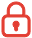订阅课程 开始学习


展开全文• 基于opencv的傅里叶变换和逆变换，有详细的代码解释。opencv
• 在matlab中对图像进行傅里叶变换和逆变换。注意：m文件中的文件路径是在本人电脑上的路径，用户应修改成在您电脑中图片的绝对路径。谢谢大家的支持！
• //快速傅里叶变换 void fft2Image(InputArray _src, OutputArray _dst) { //得到Mat类型 Mat src = _src.getMat(); //判断位深 CV_Assert(src.type() == CV_32FC1 || src.type() == CV_64FC1); CV_Assert(src....
• 根据数学分析中傅里叶级数的相关结论和黎曼积分的思想推导一维连续傅里叶变换和其逆变换的公式。
• 自己写的测试代码，里面有详细说明。不明白了可以发邮件给我，邮箱在文档里。
• 理论基础 时域：以时间为横坐标 频域：以频率的倒数为横坐标，可以看出，频域更加简单。 相位：时间差有关的一个概念。...1. 傅里叶变换 numpy.fft.fft2 1 实现傅里叶变换。 返回一个复数数组。...
• 文章目录(1)傅里叶变换(2)Graph上的傅里叶变换(3)Graph上的傅里叶逆变换总结二、为什么拉普拉斯矩阵的特征向量可以作为傅里叶变换的基？特征值表示频率？(1)为什么拉普拉斯矩阵的特征向量可以作为傅里叶变换的基？(2...
• 积分线性变换可以将信号或图像在更适合的域内表达，并且使得解决相关问题更容易，在图像分析中最常用的积分显示变换是傅里叶变换、离散余弦变换与小波变换。 1d傅里叶变换由傅里叶(Fourier)提出，1d傅里叶变换将...
• 傅里叶变换 import cv2 import numpy as np import matplotlib.pyplot as plt o=cv2.imread('image\\boat.bmp',0) #读入图片 dft=cv2.dft(np.float32(o),flags=cv2.DFT_COMPLEX_OUTPUT) ...
• 请问一个10240长的平稳序列，取前400个点做傅里叶变换，取这400个点的频谱再用逆傅里叶变换能还原出10240个点的序列数据么？ xl为10240长的序列 len(xl) = 10240 fr = fft(xl[0:400]) fr逆傅里叶变换能还原...开发语言
• 《Matlab傅里叶变换傅里叶逆变换-FFT-IFFT》由会员分享，可在线阅读，更多相关《Matlab傅里叶变换傅里叶逆变换-FFT-IFFT(2页珍藏版)》请在人人文库网上搜索。1、Matlab傅里叶变换傅里叶逆变换% 信号经过傅里叶变换...
• 代码实现了快速傅里叶变换及其逆变换的计算，幅值、初始相位的计算 import numpy as np import matplotlib.pyplot as plt Fs=500#采样频率 ts=1/Fs#采样时间间隔 L=500#信号长度 t=np.linspace(0,L-1,L)*ts x=2+0.7...
• 傅立叶变换是一种分析信号的方法，它可分析信号的成分，也可用这些成分合成信号。许多波形可作为信号的成分，比如正弦波、方波、锯齿波等...其变换与反变换 公式中F(ω)为f(t)的像函数，f(t)为F(ω)的像原函数: ...
• 【经典算法实现 40】一维傅里叶变换 及 逆变换代码实现一、一维傅里叶变换 及 其逆变换二、C代码实现 一、一维傅里叶变换 及 其逆变换 一维傅里叶变换： F(u)=∑M=0M−1f(x)e−jux2πM，u=0,1,2,⋯ ,M−1F(u) = \...
• #include #include #include #include <highgui.h>//傅里叶变换 void fft2(IplImage *src, IplImage *dst) { //实部、虚部 IplImage *image_Re = 0, *image_Im = 0, *Fourier = 0; // int iopencv...